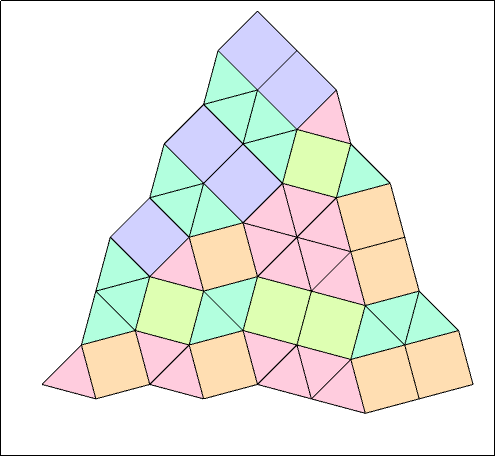# Discrete Mathematics

Listed on this page are current research projects being offered for the Vacation Scholarship Program.

## Symmetric polynomials and statistical mechanics

(Also listed in Mathematical Physics & Statistical Mechanics, posted for 2017-2018)

Symmetric polynomials are functions which exhibit symmetry under permutations of their variables. In addition to this symmetry, they usually have a host of magical properties like orthogonality relations and elegant summation identities. Despite their long history, symmetric polynomials continue to be important in many areas of contemporary mathematics including representation theory and geometry. This project will be about constructing symmetric polynomials (and understanding their properties) using exactly solvable models of statistical mechanics, and will offer a good foundation for further study in this very active subject.

Contact: Michael Wheeler, wheelerm@unimelb.edu.au

## New models of lattice polygons and polyominoes

(Also listed in Mathematical Physics & Statistical Mechanics, posted for 2017-2018)

Self-avoiding walks were originally conceived in the 1940s as a model of long polymers like DNA, but have since been the subject of many results in combinatorics, mathematical physics and probability theory, including some exciting developments in just the last few years. One useful tool is the concept of irreducible self-avoiding walks, which act a bit like the primes in number theory, and are connected to renewal processes in probability theory.

The goal of this project is to study the related concepts of irreducible polygons and polyominoes. This may involve looking at the corresponding renewal processes, doing some numerical series analysis, or using analytic combinatorics to search for solvable models.

Contact: Nicholas Beaton nrbeaton@unimelb.edu.au

## Self-interacting polymers in confinement

(Also listed in Mathematical Physics & Statistical Mechanics, posted for 2017-2018)

Self-avoiding walks on a lattice are a classical model of long polymer chains, and when equipped with a nearest-neighbour interaction energy they form a simple yet rich model of collapsing polymers. However, very little has been proven rigorously about the model in two or more dimensions.

The goal of this project is to study a simplified version of the model, namely interacting walks and/or loops in a two-dimensional strip or three-dimensional tube. These can be analysed using a transfer-matrix approach. One question is the zero-temperature limit in both the positive and negative energy regimes — that is, when very few or very many interactions are preferred. Another is the possibility of using self-interacting walks as a (highly simplified) model of strand-exchange operations in polymers like DNA, with potential applications to Monte Carlo methods for dense polymers.

Contact: Nicholas Beaton nrbeaton@unimelb.edu.au

## Schubert puzzles

(Posted for 2017-2018)

"Puzzles" are remarkable combinatorial objects that appear naturally in a branch of enumerative geometry called Schubert calculus, and in the Littlewood--Richardson rule for computing tensor products of representations of the general linear group. They have various incarnations, but can be for example depicted as square-triangle tilings of certain regions of the plane:

The goal of the project is to familiarise oneself with these combinatorial objects, and then to study variations, such as symmetry classes of puzzles, or the effect of the addition of new tiles.

Contact: Paul Zinn-Justin pzinn@unimelb.edu.au## Linear algebra and statistics

(posted for 2017-2018)

The purpose of this project is to explore how geometric quantities like angles and projection, familiar from linear algebra, show themselves in multi-dimensional statistics

Contact: Peter Forrester pjforr@unimelb.edu.au

## Continued fractions

(posted for 2017-2018)

The mathematics underlying continued fractions shows itself in many different sub-disciplines, for example number theory, dynamical systems and the analysis of algorithms. After some familiarising with the classical theory, the project aims to consider the less familiar complex case, where the numbers appearing are Gaussian integers.

Contact: Peter Forrester pjforr@unimelb.edu.au

## Perfect codes in Cayley graphs

(Also listed in Operations Research, posted for 2016-2017)

Perfect codes have been important objects of study ever since the dawn of coding theory in the late 1940s, and after more than six decades they still receive much attention today. The aim of this project is to study perfect codes in Cayley graphs, which are generalizations of perfect codes in the classical setting.

Contact: Sanming Zhou sanming@unimelb.edu.au

## Arc-transitive graphs

(Also listed in Operations Research, posted for 2016-2017)

A finite graph is a finite set together with a family of 2-element subsets of the set; each element in the set is called a vertex and each 2-element subset in the family is called an edge. An oriented edge is called an arc. If all vertices have the “same status” in the graph and all arcs have the “same status” as well, then the graph is said to be arc-transitive. Examples of arc-transitive graphs include the 1-skeletons of the five Platonic polyhedral. The study of arc-transitive graphs has long been an important area in algebraic graph theory with strong links to group theory. The goal of this project is to study some families of arc-transitive graphs whose automorphism groups contain a subgroup acting imprimitively on the vertex set.

Contact: Sanming Zhou sanming@unimelb.edu.au

## Cores of graphs

(Also listed in Operations Research, posted for 2016-2017)

A homomorphism from a graph X to a graph Y is a mapping from the vertex set of X to the vertex set of Y that preserves the adjacency relation. Two graphs X, Y are said to be homomorphically equivalent if there is a homomorphism from X to Y and also there is a homomorphism from Y to X. The smallest induced subgraph of X that is homomorphically equivalent to X is called the core of X. This notion plays an important role in understanding structure and colouring of graphs. It is also closely related to several application domains, including synchronization. The purpose of this project is to understand the cores of some families of graphs, especially Cayley graphs of some finite groups.

Contact: Sanming Zhou sanming@unimelb.edu.au